How Cheenta works to ensure student success?
Explore the Back-Story

# Definite Integral | IIT JAM 2018 | Problem 4Try this beautiful problem from IIT JAM 2018 which requires knowledge of the properties of Definite integral.

## Properties of Definite Integral -IIT JAM2018 (Problem 4)

Let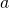be positive real number. If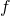is a continuous and even function defined on the interval, then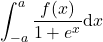is equal to :-

•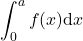••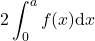•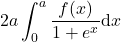### Key Concepts

Definite Integral

Properties of definite Integral

Even function / Odd function

Answer:IIT JAM 2018, Problem 4

Definite and Integral calculus : R Courant

## Try with Hints

In this first I will give you the properties we need to solve this problem :

Property 1 :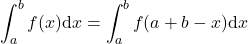[Whereis continuous on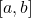]

Property 2 : Ifis an even function i.e.,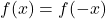then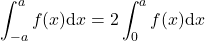Can you drive it from here !!!! Give it a try !!!

Let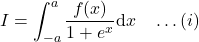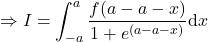[Since,is continuous then]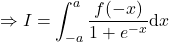[Since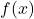is even]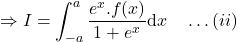Adding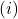and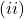we can get some interesting result !!!

Addingandwe get ,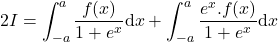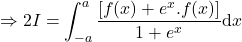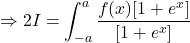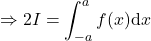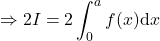[Sinceis even ]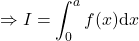[ANS]

## Subscribe to Cheenta at Youtube

Try this beautiful problem from IIT JAM 2018 which requires knowledge of the properties of Definite integral.

## Properties of Definite Integral -IIT JAM2018 (Problem 4)

Letbe positive real number. Ifis a continuous and even function defined on the interval, thenis equal to :-

••••### Key Concepts

Definite Integral

Properties of definite Integral

Even function / Odd function

Answer:IIT JAM 2018, Problem 4

Definite and Integral calculus : R Courant

## Try with Hints

In this first I will give you the properties we need to solve this problem :

Property 1 :[Whereis continuous on]

Property 2 : Ifis an even function i.e.,thenCan you drive it from here !!!! Give it a try !!!

Let[Since,is continuous then][Sinceis even]Addingandwe can get some interesting result !!!

Addingandwe get ,[Sinceis even ][ANS]

## Subscribe to Cheenta at Youtube

This site uses Akismet to reduce spam. Learn how your comment data is processed.

### Knowledge Partner